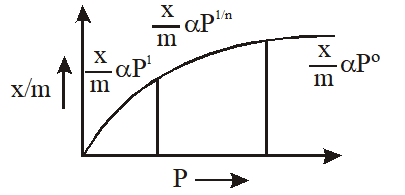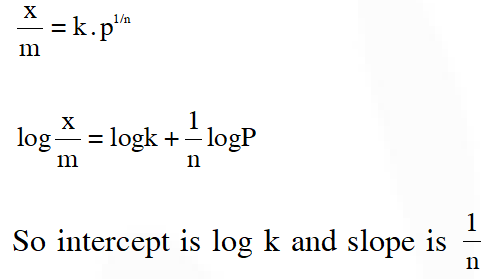Download the eSaral app and start learning from Kota's top IITians and doctors.

# Surface Chemistry - JEE Main Previous Year Questions with Solutions

JEE Main Previous Year Papers Questions of Chemistry with Solutions are available at eSaral. Practicing JEE Main chapter wise questions of Chemistry will help the JEE aspirants in realizing the question pattern as well as help in analyzing weak & strong areas.   Simulator   Previous Years AIEEE/JEE Main Questions
Q. Which of the following statements is incorrect regarding physisorptions ? (1) Under high pressure it results into multi molecular layer on adsorbent surface (2) Enthalpy of adsorption $\left(\Delta \mathrm{H}_{\text {adsorption }}\right)$ is low and positive (3) It occurs because of Van der Waal's forces (4) More easily liquefiable gases are adsorbed readi AIEEE-2009
Ans. (2) $\Delta \mathrm{H}$ is negative
Q. According to Freundlich adsorption isotherm, which of the following is correct ? (1) $\frac{\mathrm{x}}{\mathrm{m}} \propto \mathrm{p}^{0}$ (2) $\frac{\mathrm{x}}{\mathrm{m}} \propto \mathrm{p}^{1}$ (3) $\frac{\mathrm{x}}{\mathrm{m}} \propto \mathrm{p}^{1 / \mathrm{n}}$ (4) All the above are correct for different ranges of pressure AIEEE-2012
Ans. (4)Q. The coagulating power of electrolytes having ions $\mathrm{Na}^{+}, \mathrm{Al}^{3+}$ and $\mathrm{Ba}^{2+}$ for aresenic sulphide sol increases in the order :- (1) $\mathrm{Al}^{3+}<\mathrm{Ba}^{2+}<\mathrm{Na}^{+}$ (2) $\mathrm{Na}^{+}<\mathrm{Ba}^{2+}<\mathrm{Al}^{3+}$. (3) $\mathrm{Ba}^{2+}<\mathrm{Na}^{+}<\mathrm{Al}^{3+}$ (4) $\mathrm{Al}^{3+}<\mathrm{Na}^{+}<\mathrm{Ba}^{2+}$ JEE-Main 2013
Ans. (2) According to hardley schuzle rule
Q. For a linear plot of log(x/m) versus log p in a Freundlich adsorption isotherm, which of the following statements is correct ? (k and n are constants) (1) log (1/n) appears as the intercept (2) Both k and 1/n appear in the slope term (3) 1/n appears as the intercept (4) Only 1/n appears as the slope JEE-Main 2016
Ans. (4) According to Freundlich isothermQ. The Tyndall effect is observed only when following conditions are satisfied :- (a) The diameter of the dispersed particles is much smaller than the wavelength of the ligh used. (b) The diameter of the dispersed particle is not much smaller than the wavelength of the light used. (c) The refractive indices of the dispersed phase and dispersion medium are almost similar in magnitude. (d) The refractive indices of the dispersed phase and dispersion medium differ greatly in magnitude. (1) (a) and (d) (2) (b) and (d) (3) (a) and (c) (4) (b) and (c) JEE - Main - 2017
Ans. (2) As per NCERT book (fact)

Bunty
Aug. 16, 2021, 2:54 p.m.
Divyansh
Feb. 27, 2021, 11:01 a.m.
bhavana
Jan. 28, 2021, 10:22 p.m.
good effort
GB
Sept. 4, 2020, midnight